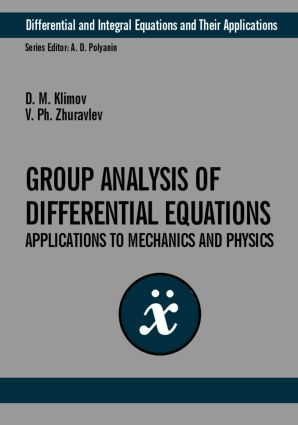# Group-Theoretic Methods in Mechanics and Applied Mathematics

## 1st Edition

CRC Press

240 pages

Hardback: 9780415298636
pub: 2002-08-15
SAVE ~\$29.00
\$145.00
\$116.00
x

FREE Standard Shipping!

### Description

Group analysis of differential equations has applications to various problems in nonlinear mechanics and physics. For the first time, this book gives the systematic group analysis of main postulates of classical and relativistic mechanics. The consistent presentation of Lie group theory is illustrated by plentiful examples. Symmetries and conservation laws of differential equations are studied. Specific equations and problems of mechanics and physics are considered, and exact solutions are given for the following equations: dynamics of rigid body, heat transfer, wave, hydrodynamics, Thomas-Fermi and more. The author pays particular attention to the application of group analysis to developing asymptotic methods of applied mathematics in problems with small parameter. The methods are used to solve basic equations (Van Der Pol's equation, Duffing equation, etc.) encountered in the theory of nonlinear oscillations. This book is intended for a wide range of scientists, engineers and students in the fields of applied mathematics, mechanics and physics.

Annotation Preface. Basic Notions of Lie Groups. Group Analysis of Basic Postulates of Classical and Relativistic Mechanics. Fundamental Theorems and Conservation Laws. Applications of Group Analysis to Problems of Mechanics and Physics. Construction of Asymptotic Expansions with the Aid of Group Methods. Nonlinear Problems in Theory of Oscillations References. Index.